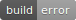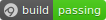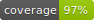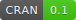# bindrActive bindings in R are much like properties in other languages: They look like a variable, but querying or setting the value triggers a function call. They can be created in R via `makeActiveBinding()`, but with this API the function used to compute or change the value of a binding cannot take additional arguments. The `bindr` package faciliates the creation of active bindings that are linked to a function that receives the binding name, and an arbitrary number of additional arguments.

## Installation

You can install `bindr` from GitHub with:

``````# install.packages("devtools")
devtools::install_github("krlmlr/bindr")``````

## Getting started

For illustration, the `append_random()` function is used. This function appends a separator (a dash by default) and a random letter to its input, and talks about it, too.

``````set.seed(20161510)
append_random <- function(x, sep = "-") {
message("Evaluating append_random(sep = ", deparse(sep), ")")
paste(x, sample(letters, 1), sep = sep)
}

append_random("a")
#> Evaluating append_random(sep = "-")
#>  "a-k"
append_random("X", sep = "+")
#> Evaluating append_random(sep = "+")
#>  "X+u"``````

In this example, we create an environment that contains bindings for all lowercase letters, which are evaluated with `append_random()`. As a result, a dash and a random letter are appended to the name of the binding:

``````library(bindr)
env <- create_env(letters, append_random)
ls(env)
#>   "a" "b" "c" "d" "e" "f" "g" "h" "i" "j" "k" "l" "m" "n" "o" "p" "q"
#>  "r" "s" "t" "u" "v" "w" "x" "y" "z"
env\$a
#> Evaluating append_random(sep = "-")
#>  "a-p"
env\$a
#> Evaluating append_random(sep = "-")
#>  "a-j"
env\$a
#> Evaluating append_random(sep = "-")
#>  "a-b"
env\$c
#> Evaluating append_random(sep = "-")
#>  "c-b"
env\$Z
#> NULL``````

Bindings can also be added to existing environments:

``````populate_env(env, LETTERS, append_random, "+")
env\$a
#> Evaluating append_random(sep = "-")
#>  "a-z"
env\$Z
#> Evaluating append_random(sep = "+")
#>  "Z+j"``````

## Further properties

Both named and unnamed arguments are supported:

``````create_env("binding", paste, "value", sep = "-")\$binding
#>  "binding-value"``````

A parent environment can be specified for creation:

``````env2 <- create_env("a", identity, .enclos = env)
env2\$a
#> a
env2\$b
#> NULL
get("b", env2)
#> Evaluating append_random(sep = "-")
#>  "b-m"``````

``````create_local_env <- function(names) {
paste_with_dash <- function(...) paste(..., sep = "-")
binder <- function(name, append) paste_with_dash(name, append)
create_env(names, binder, append = "appending")
}

env3 <- create_local_env("a")
env3\$a
#>  "a-appending"``````

``````env3\$a <- NA
env3\$a <- NULL

Existing variables or bindings are not overwritten:

``````env4 <- as.environment(list(a = 5))
populate_env(env4, list(quote(b)), identity)
ls(env4)
#>  "a" "b"
populate_env(env4, letters, identity)
#> Error in populate_env(env4, letters, identity): Not creating bindings for existing variables: b, a``````

## Active bindings and C++

Active bindings must be R functions. To interface with C++ code, one must bind against an exported Rcpp function, possibly with `rng = false` if performance matters. The `bindrcpp` package uses `bindr` to provide an easy-to-use C++ interface for parametrized active bindings, and is the recommended way to interface with C++ code. In the remainder of this section, an alternative using an exported C++ function is shown.

The following C++ module exports a function `change_case(to_upper = FALSE)`, which is bound against in R code later.

``````#include <Rcpp.h>

#include <algorithm>
#include <string>

using namespace Rcpp;

// [[Rcpp::export(rng = FALSE)]]
SEXP change_case(Symbol name, bool to_upper = false) {
std::string name_string = name.c_str();
std::transform(name_string.begin(), name_string.end(),
name_string.begin(), to_upper ? ::toupper : ::tolower);
return CharacterVector(name_string);
}``````

Binding from R:

``````env <- create_env(list(as.name("__ToLower__")), change_case)
populate_env(env, list(as.name("__tOuPPER__")), change_case, TRUE)
ls(env)
#>  "__ToLower__" "__tOuPPER__"
env\$`__ToLower__`
#>  "__tolower__"
get("__tOuPPER__", env)
#>  "__TOUPPER__"``````